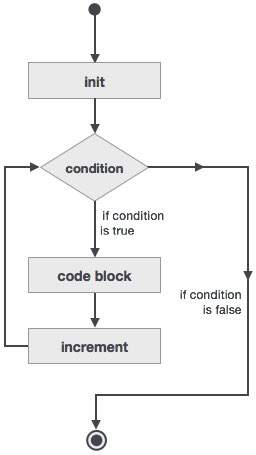# Fortran - Do Loop Construct

The do loop construct enables a statement, or a series of statements, to be carried out iteratively, while a given condition is true.

## Syntax

The general form of the do loop is −

```do var = start, stop [,step]
! statement(s)
…
end do
```

Where,

• the loop variable var should be an integer

• start is initial value

• stop is the final value

• step is the increment, if this is omitted, then the variable var is increased by unity

## For example

```! compute factorials
do n = 1, 10
nfact = nfact * n
! printing the value of n and its factorial
print*,  n, " ", nfact
end do
```

## Flow Diagram

Here is the flow of control for the do loop construct −

• The initial step is executed first, and only once. This step allows you to declare and initialize any loop control variables. In our case, the variable var is initialised with the value start.

• Next, the condition is evaluated. If it is true, the body of the loop is executed. If it is false, the body of the loop does not execute and flow of control jumps to the next statement just after the loop. In our case, the condition is that the variable var reaches its final value stop.

• After the body of the loop executes, the flow of control jumps back up to the increment statement. This statement allows you to update the loop control variable var.

• The condition is now evaluated again. If it is true, the loop executes and the process repeats itself (body of loop, then increment step, and then again condition). After the condition becomes false, the loop terminates.## Example 1

This example prints the numbers 11 to 20 −

```program printNum
implicit none

! define variables
integer :: n

do n = 11, 20
! printing the value of n
print*,  n
end do

end program printNum
```

When the above code is compiled and executed, it produces the following result −

```11
12
13
14
15
16
17
18
19
20
```

## Example 2

This program calculates the factorials of numbers 1 to 10 −

```program factorial
implicit none

! define variables
integer :: nfact = 1
integer :: n

! compute factorials
do n = 1, 10
nfact = nfact * n
! print values
print*,  n, " ", nfact
end do

end program factorial
```

When the above code is compiled and executed, it produces the following result −

```1             1
2             2
3             6
4            24
5           120
6           720
7          5040
8         40320
9        362880
10       3628800
```
fortran_loops.htm